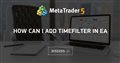# Opening Positions only between 3 a.m and 9 a.m

I am learning MQL5,

i have a small expert that buys with a tp and sl,

i want the ea to buy only when the time is between input time 1 and input time 2,
it s simple, you only have 1 trade at a time and once closed by tp or sl open a new one if the time is still in the range

but still the ea is buying in all hours of the day
need help
this is the code:

```#include <Trade\Trade.mqh>

input double SL_Levels=20;

input double TP_Levels=10;

input double Lot_Size=0.01;

input ulong MagicNumber=678910;

//input datetime starttime = D'02:00:00';

//input datetime endtime = D'22:00:00';

// local variables

double OrderPrice;

double ShortPrice;

double Stop;

double Target;

int PipValue=1;

void OnTick()

{

if(checktime("02:00:00","06:00:00")){

Process();

}

}

void Process()

{

double Bid = SymbolInfoDouble(Symbol(), SYMBOL_BID);

int Count = FindPositions();

if(Count==0)

{

}

{

OrderPrice=0.0;

ShortPrice=0.0;

}

}

int FindPositions()

{

int found=0;

for(int i=PositionsTotal()-1; i>=0; i--)

{

if(PositionSelect(Symbol()))

if(PositionGetInteger(POSITION_MAGIC) == MagicNumber)

{

found++;

}

}

return(found);

}

{

bool ot;

bool res=false;

ulong ticket = 0;

if (checktime("02:00:00","12:00:00")){

}

if(ot)

{

do

while(!PositionSelectByTicket(ticket));

OrderPrice=PositionGetDouble(POSITION_PRICE_OPEN);

ShortPrice=OrderPrice - (Level_Size*HedgeLvl*PipValue*Point());

Stop=OrderPrice - (SL_Levels*Level_Size*PipValue*Point());

Target=OrderPrice + (TP_Levels*Level_Size*PipValue*Point());

}

return(res);

//   }

//    return true;

}

//+------------------------------------------------------------------+

//|                                                                  |

//+------------------------------------------------------------------+

void CloseAll()

{

for(int i=PositionsTotal()-1; i>=0; i--)

{

if(PositionSelect(Symbol()))

// position with appropriate ORDER_MAGIC, symbol and order type

if(PositionGetInteger(POSITION_MAGIC) == MagicNumber)

{

ulong tic = PositionGetInteger(POSITION_TICKET);

}

}

}

}```

Time Filter Code Sample =>how can i add timefilter in eahow can i add timefilter in ea
• 2020.02.14
• www.mql5.com
can someone help me to add time filter in my ea...

Here's a quick and simple solution:

```datetime dtnow=TimeCurrent();

if(dtnow>=StringToTime("03:00") && dtnow<StringToTime("09:00"))
{
Process();
}```
Reason: